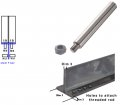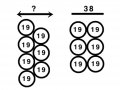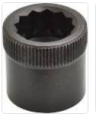# Calculation of dimensions

#### Andersons

Joined Sep 28, 2019
24
Hello, I’m calculating dimensions of threaded rods that will be attached to a steel T bar. It’s important that I get everything right, so I thought that consultation might be a good idea.

What I’m doing is best described in pictures.The diameter of the rods is 19 millimeter. The diameter of the threading of the rod is M8. The nuts I’ll use is these http://www.fasteners.eu/standards/DIN/934/ The value ”e” describe the overall diameter of the nuts, they only tell the ”minimum”, but I need to now the maximum.. Anyway, I try to calculate how much space will be left for the nut if the T bar is 3.5mm thick (as described in figure: Dim 3 right bottom corner). The (minimum) total diameter of the nut is 14.38mm according to DIN 934, so I need to have atleast 16mm as a minimum marginal space.

The reason why the rods in the 2-D image in the left corner (seen from above) overlap eachother is this:The figure to the left is how the rods are assembled. What’s the total diameter of the figure to the left?

Last edited:

#### KeithWalker

Joined Jul 10, 2017
1,216
We do not do the calculations for you. If you show us your best effort, we may be able to offer some advice.
I will give you a clue: In the bottom left illustration, the centers of every three adjacent rods forms an equilateral triangle. Can you take it from there?
Regards,
Keith

•sagor

#### WBahn

Joined Mar 31, 2012
26,140
What does the specified dimensions of s tell you about the max value of e?

#### hrs

Joined Jun 13, 2014
251
Is this an exercise in geometry or something that actually needs to be made? Bolt spacing is typically limited by tooling and 19 mm seems marginal.

#### jpanhalt

Joined Jan 18, 2008
10,210
If you space the nuts to assure no interference by dimension "e" of the hex nut, they will be difficult to tighten, as the wrench is even wider. You can make a nut driver (socket) and turn it to a smaller outside diameter. There are "Allen" nuts that can be spaced closer together and tightened with a spline wrench from the top:You can weld the nut and try to tighten the rod, or thread the hole and tighten the rod. There are other possibilities along that line too. Tightening a smooth round rod is can be difficult.

Assuming you want to tighten a conventional nut of about the same size on the rod, I would not pack the rods so tightly together. The rod can be turned smaller and threaded. Then use a smaller nut. There are also small outline nuts made.

Bottom line: You need to decide how many rods you want, the type of nut you will use, if any, and from that calculate the minimal spacing with allowance for a wrench used to tighten them.

Edit: You can make it easier on yourself by drawing nuts with concentric circles showing maximum diameter of the head and tightening wrench.

Last edited:

#### Wendy

Joined Mar 24, 2008
22,164
I recently solved a similar issue laying out a 3D print. It is done using radius and Pythagorean theorem ( right angles).

#### KeithWalker

Joined Jul 10, 2017
1,216
So the total diameter of the left figure is 33mm?
In planar geometry, only a circle has a diameter. The figure in the diagram on the left has width and height.
The answer that you calculated, 33mm, is the distance between the parallel lines which pass vertically through the centers of the two columns of circles. The total width of the diagram is 33mm + (R X 2) where R is the radius of the circles.
You need to be very specific when you are discussing measurements and calculations, otherwise you comments are meaningless.
Regards,
Keith